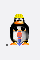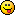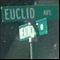Discussion about math, puzzles, games and fun.   Useful symbols: ÷ × ½ √ ∞ ≠ ≤ ≥ ≈ ⇒ ± ∈ Δ θ ∴ ∑ ∫  π  -¹ ² ³ °

You are not logged in.

## #26 2006-07-24 01:24:29

ben
Member
Registered: 2006-07-12
Posts: 106

### Re: An abstract introduction to Groups and Fields.

ben wrote:

The proof that inverses are identically left and right is a bit more tedious, but equally trivial. If anyone wants a crack, remember that (a^-1)^-1 = a.

Well you guys are no fun! Here.

Let

be a right inverse. We know that ae = a. Note first that
.

by associativity

This suggests that last term is a right inverse

But

by assumption, so I have

TraLa!

Offline

## #27 2006-08-01 04:22:53

ben
Member
Registered: 2006-07-12
Posts: 106

### Re: An abstract introduction to Groups and Fields.

ben wrote:

To
formalize: given a normal subgroup A of a group G, gh^-1 is in A
defines and equivalence relation ~ on G iff the relation is
reflexive: g ~ g
symmetric: g ~ h ==> h ~ g
transitive: g ~ h and h ~ k ==> g ~ k
for all g, h, k in G.
This is an example of a universal construction, and is highly
important. This post is already over long. More later, if anybody
wants.

That's where I left off last time. Let me continue (even though there
doesn't seem a lot of interest. Do you all know this stuff already? Why no challenges? Too easy? Huh?)

Using the notation in my self-quote, if g ~ h ~ k, we may form an
equivalence class {g, h, k}, for which we can elect a class
representative, say g. Then we write that class as [g], it being
understood that g, h and k are in [g].

Note that, by the property of transitivity above, any element g of G
can only be in one class: if g is in [g] and g is in [j], then [g] =
[j]. This is thus a partition of G (strictly of the underlying set |G|) into
disjoint subsets. Here's the nifty bit. Ah no, wait, let's get concrete
(but remember the caveat I gave when introducing the permutation
groups).

I talked about the normal subgroup 3Z of Z, that being the
elements of Z exactly divisible by 3. We saw (at least I did!) that 1 ~
4 ~ 7 (for 1 + 6 = 4 + 3......) and {2 ~ 5 ~ 8, and we (!) noted that
not only did elements of 3Z differ by a factor of 3, so did the
elements of the sets {1, 4, 7...} and 2, 5, 8....}. In fact I think I
presented that the wrong way round, but you get the picture.

So we have 3 equivalence classes: ,  and . But wait! The
subgroup 3Z has as elements {...-6, -3, 0, 3, 6,...} so let's run the
election for class representative again: ,  and , let's say.
So if we gather these guys together we have an identity in . We also know that any member of  plus any member of  is a member of  etc. so we have a closed operation too. Inverses are similarly easily checked.

In other words, the collection of equivalence classes is itself a group
which satisfies all the usual group axioms. Its called the quotient
group. It is written G/~. That's the nifty bit I referred to earlier.

To sum up. For any group G, the set comprising a normal subgroup H of G together with its cosets forms a group, the quotient group G/~. This is an incredibly important construction, and not only in group theory.

Got to run now, but next up (if you want it): groups talk to each other! Wow!

Offline

## #28 2006-08-01 06:41:10

numen
MemberRegistered: 2006-05-03
Posts: 115

### Re: An abstract introduction to Groups and Fields.

Hmm... I think I need to read all this again, now I see why it's called an abstract introductionI think groups, fields and rings will be introduced in about 2-3 years from where I am now, so I don't have to read everything that fast, hehe.

Bang postponed. Not big enough. Reboot.

Offline

## #29 2006-08-09 03:52:33

ben
Member
Registered: 2006-07-12
Posts: 106

### Re: An abstract introduction to Groups and Fields.

In the hope that Ricky's introduction to groups on the main page may have stimulated some interest in this subject, I'll plod on (not hopefully, though).

I ended my last with the remark that groups can talk to each other.
That was, of course, a bit of hyperbole.. So what did I mean? Here....
To each group G we can associate its underlying set, which I earlier
denoted |G|. Now we know that functions on sets take elements of
one set to elements of another thusly: f: X → Y. Let's quickly remind ourselves about such functions. (By the way, I use the terms function and map interchangably, I hope that's OK with you)

If a function f: X → Y is such that for every y in Y there is at
least
one x in X such that f(x) = y, we say that f is surjective
(or onto). And if for each y in Y there is at most one x in X
for which f(x) = y we say f is injective (or one-to-one). Just note that for a surjection there are no elements in the codomain which are not mapped onto by element(s) of the domain, while for an injection there are no elements in the domain which share an image in the codomain

Now, given a function on |G|, we may have that h: |G| → |H|, where |H| is the underlying set of some group H. But groups carry a notion of structure, built on the allowable group operation and the axioms which provide the "rules of manipulation" of group objects. So we require our function h to be rather more that a function on sets.

Let's have now f: G → H. If f preserves the operational relationship
between elements of G and H and is surjective, it is called a
homomorphism. Specifically, let the operation on G be × and that on H be +, we will have f(g × g) = f(g) + f(g) for all g in G and all f(g)
in H. Here's a famliar example. log(xy) = log(x) + log(y). This is in fact an example of an injective function, that is if f: G → H is injective f is said to be an isomorphism: a one-to-one, operation-preserving map.

OK. (You at the back, are you paying attention?) One of the most important theorems in group theory follows. Remember that both Ricky and I talked about the permutation (a.k.a the symmetric) groups? Some cool fella called Cayley came up with this:

Every group of order n is isomorphic to a subgroup of the symmetric group S_n (order n!)

The proof is straightforward, but rather messy. Anyway, think about it in the conext of the theorem of Lagrange that I gave earlier. I'll show it if you want.

Offline

## #30 2006-08-09 04:19:55

Ricky
ModeratorRegistered: 2005-12-04
Posts: 3,791

### Re: An abstract introduction to Groups and Fields.

One of the most important theorems in group theory follows.

That should have read:

One of the most important, and useless, theorems in group theory follows.

Cayley's theorem has no pratical value.  It tells us that we can know of, and represent, every group that can ever exist (unlike some other things in math, for example, some real numbers are transendental).  Other than that, it can't be used for really anything.

But even though we can't get any practical uses out of it, it is still very important.  It's as if you were lead up to the top of a mountain, and down below, you can see your kindom of groups.  You can literally see everything.

"In the real world, this would be a problem.  But in mathematics, we can just define a place where this problem doesn't exist.  So we'll go ahead and do that now..."

Offline

## #31 2006-08-09 04:35:38

ben
Member
Registered: 2006-07-12
Posts: 106

### Re: An abstract introduction to Groups and Fields.

Ricky wrote:

Cayley's theorem has no pratical value.  It tells us that we can know of, and represent, every group that can ever exist (unlike some other things in math, for example, some real numbers are transendental).  Other than that, it can't be used for really anything.

And you really believe this is a trivial result? Wait till you do the fundamental group of Poincaré, or Lie groups, for that matter.

Don't be so rude about Cayley. Grr.

Offline

## #32 2006-08-09 04:58:44

Ricky
ModeratorRegistered: 2005-12-04
Posts: 3,791

### Re: An abstract introduction to Groups and Fields.

Not that it's trivial, just that it has no pratical applications.

"In the real world, this would be a problem.  But in mathematics, we can just define a place where this problem doesn't exist.  So we'll go ahead and do that now..."

Offline

## #33 2006-08-09 06:37:28

ben
Member
Registered: 2006-07-12
Posts: 106

### Re: An abstract introduction to Groups and Fields.

Then I don't understand what you mean by practical applications. In my mind, a unifying theory that brings all sorts of groups together, in some sense, is of immense value. Most of the really important stuff in math that I can bring to mind falls into that category.

Maybe you mean, Cayley has no applications in physics?

Last edited by ben (2006-08-09 06:38:27)

Offline

## #34 2006-08-09 07:05:27

Ricky
ModeratorRegistered: 2005-12-04
Posts: 3,791

### Re: An abstract introduction to Groups and Fields.

Many proofs require the use of the Euclidean algorithm, or Langrange's theorem, or any other theorem.  What proofs use Cayley's theorem?  That's what I mean by no practical use.

"In the real world, this would be a problem.  But in mathematics, we can just define a place where this problem doesn't exist.  So we'll go ahead and do that now..."

Offline

## #35 2006-08-09 09:10:51

sk
Member
Registered: 2006-08-04
Posts: 19

### Re: An abstract introduction to Groups and Fields.

I am very much interested in Astronomy. Suggest me some good books to know deep about and on Astronomy.

Offline

## #36 2006-08-09 09:12:33

ben
Member
Registered: 2006-07-12
Posts: 106

### Re: An abstract introduction to Groups and Fields.

Ricky -  I must say I find your utilitarian take on math rather depressing. Surely, there are some theorems that have a beauty in their own right, irrespective of their ability to prove other theorems?

Anyway look. I don't seem able to get a discussion going on group theory, so I'll reluctantly leave it. I was sure there were questions to be asked, but none came. No interest all round, it seems. Oh well.

Last edited by ben (2006-08-09 09:13:32)

Offline

## #37 2006-08-09 09:32:17

Ricky
ModeratorRegistered: 2005-12-04
Posts: 3,791

### Re: An abstract introduction to Groups and Fields.

Ben, I'm guessing you didn't read this part of my post:

But even though we can't get any practical uses out of it, it is still very important.  It's as if you were lead up to the top of a mountain, and down below, you can see your kindom of groups.  You can literally see everything.

"In the real world, this would be a problem.  But in mathematics, we can just define a place where this problem doesn't exist.  So we'll go ahead and do that now..."

Offline

## #38 2006-08-09 09:41:23

Ricky
ModeratorRegistered: 2005-12-04
Posts: 3,791

### Re: An abstract introduction to Groups and Fields.

As for a discussion of Group theory, I found my self wondering today, "What is a homomorphism?"  Not the definition.  Not what you can use it for.  But in a very abstract way, what is it?

For example, isomorphisms are easy, they are what you use to say that two groups are equal.  But is there a way we can use that kind of language with homomorphisms?

One thing I thought of, is that a homomorphism tells you that two groups act the same, even if they have a different order.  With this, I'm tempted to say that homomorphisms are group equality, rather than isomorphisms.  What do you think?

"In the real world, this would be a problem.  But in mathematics, we can just define a place where this problem doesn't exist.  So we'll go ahead and do that now..."

Offline

## #39 2006-08-09 22:12:30

ben
Member
Registered: 2006-07-12
Posts: 106

### Re: An abstract introduction to Groups and Fields.

Ricky wrote:

One thing I thought of, is that a homomorphism tells you that two groups act the same, even if they have a different order.

If by "act" in the forgoing you mean that algebraic structure is preserved, yes I agree

With this, I'm tempted to say that homomorphisms are group equality, rather than isomorphisms.  What do you think?

No, I don't think that's right. Bear in mind that all groups are homomorphic to the trivial group 1 = {e}. Preservation of group structure follows trivially from the definition of the identity, but we would scarely be tempted to say that all groups are equal to {e}!

By the way, I'm sure you know this Ricky, but others may not. There is a useful jargon phrase "up to", as in G = H up to isomorphism, for example. This means that for the purpose in hand, we can regard G and H as the same, even though they are in reality isomorphic rather than strictly equal.

Equality in the group theory sense means much the same as anywhere else: the underlying sets are equal, the operation is the same, the axioms are the same etc.

Offline

## #40 2006-08-10 02:27:40

Ricky
ModeratorRegistered: 2005-12-04
Posts: 3,791

### Re: An abstract introduction to Groups and Fields.

Good point.

As for isomorphims and group equality, when dealing with them as groups and only groups, the only real difference between two isomorphic groups is that the symbols change.  Everything else, from within a group sense, stays the same.  It's when you go outside of group theory with groups that you need a stricter meaning of "equality".

"In the real world, this would be a problem.  But in mathematics, we can just define a place where this problem doesn't exist.  So we'll go ahead and do that now..."

Offline

## #41 2006-08-10 03:02:09

ben
Member
Registered: 2006-07-12
Posts: 106

### Re: An abstract introduction to Groups and Fields.

Well yes and no. Remember (why should you) the example I gave: log(xy) = log(x) + log(y)? Let f(x) = log(x), this becomes f(x·y) = f(x) + f(y), so f is an isomorphism.
You see it's not so much a matter of re-naming the group elements, it can be shuffling them around (basically we learn this from Dr Cayley) and (possibly) changing the operation, as above, provided that the equality f(x·y) = f(x)*f(y) holds - here the centre dot and the star can be any operation we choose.

But yes, group theorists don't get too exercised about the difference between say, {0,1} and {-1,+1}. They are clearly equal only "up to isomorphism" , but that's usually all these guys care about.

On a slightly deeper note: strict equality is devilish hard to pin down. In what sense, say, is the abstraction 1 + 1 identically the same as the abstraction 2. I come from alpha centuri, I can see they are not the same objects, but you might convince me they are isomorphic!!!

Offline

## #42 2017-04-29 09:55:45

zetafunc
ModeratorRegistered: 2014-05-21
Posts: 2,249
Website

### Re: An abstract introduction to Groups and Fields.

Ricky wrote:

For example, isomorphisms are easy, they are what you use to say that two groups are equal.  But is there a way we can use that kind of language with homomorphisms?

Aside from preserving an algebraic structure, there are ways in which such algebraic structures can be visualised. One of my favourite examples includes homomorphisms of directed graphs.

Offline